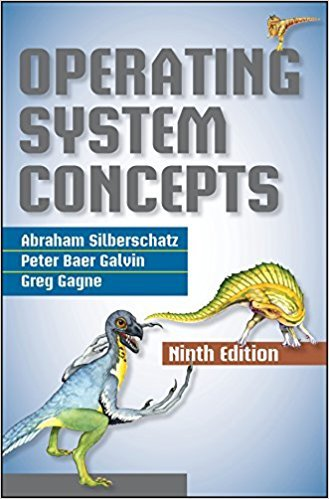×
×

# Consider the exponential average formula used to predict the length ofthe next CPUISBN: 9781118063330 327

## Solution for problem 6.14 Chapter 6

Operating System Concepts | 9th Edition

• Textbook Solutions
• 2901 Step-by-step solutions solved by professors and subject experts
• Get 24/7 help from StudySoup virtual teaching assistantsOperating System Concepts | 9th Edition

4 5 1 425 Reviews
10
5
Problem 6.14

Consider the exponential average formula used to predict the length ofthe next CPU burst. What are the implications of assigning the followingvalues to the parameters used by the algorithm?a. = 0 and 0 = 100 millisecondsb. = 0.99 and 0 = 10 milliseconds

Step-by-Step Solution:
Step 1 of 3

Microscopic structure of skeletal muscle tissue 1. Skeletal muscle is composed of thousands or multinucleated, long, cylindrical cells known as muscle fibers. 2. These fibers lie parallel to each other which can be referred to as bundled. 1. The muscle...

Step 2 of 3

Step 3 of 3

##### ISBN: 9781118063330

Since the solution to 6.14 from 6 chapter was answered, more than 209 students have viewed the full step-by-step answer. The full step-by-step solution to problem: 6.14 from chapter: 6 was answered by , our top Science solution expert on 03/05/18, 08:03PM. This textbook survival guide was created for the textbook: Operating System Concepts , edition: 9. The answer to “Consider the exponential average formula used to predict the length ofthe next CPU burst. What are the implications of assigning the followingvalues to the parameters used by the algorithm?a. = 0 and 0 = 100 millisecondsb. = 0.99 and 0 = 10 milliseconds” is broken down into a number of easy to follow steps, and 43 words. Operating System Concepts was written by and is associated to the ISBN: 9781118063330. This full solution covers the following key subjects: . This expansive textbook survival guide covers 20 chapters, and 499 solutions.

Unlock Textbook Solution